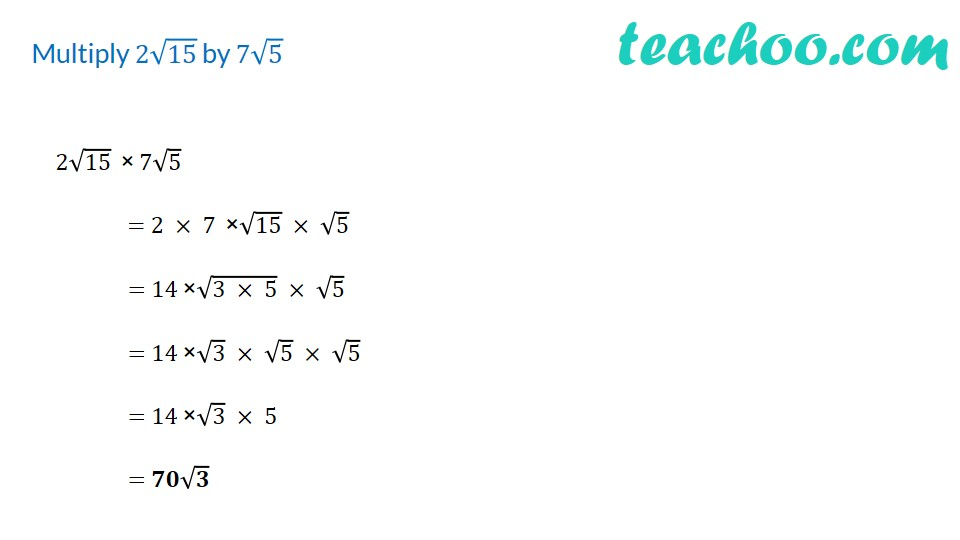1. Chapter 1 Class 9 Number Systems (Term 1)
2. Concept wise
3. Rationalising

Transcript

Multiply 2√15 by 7√5 2√15 × 7√5 = 2 × 7 ×√15 × √5 = 14 ×√(3 × 5) × √5 = 14 ×√3 × √5 × √5 = 14 ×√3 × 5 = 𝟕𝟎√𝟑

Rationalising